# Solving Pattern Programs - Part 2

## ICSE Computer Applications

This lesson builds upon the pattern programming concepts we learnt in the previous lesson. It will show you how to write Java programs for Right-Angled Triangle patterns. In case you have not yet watched the previous lesson of solving pattern programs, I strongly recommend you watch it and then start with this lesson.

## Pattern 1

Let’s start by solving this pattern:

*
* *
* * *
* * * *

See these stars are forming a right-angled triangle. That’s why such patterns are called right-angled triangle based patterns. Like rectangular patterns, here also we will start by looking at the three basic things of the pattern that are:

1. Number of rows
2. Number of terms in each row
3. What is getting printed in the pattern.

This pattern has 4 rows. So, the outer loop will run four times from 1 to 4 like this — `for (int i = 1; i <= 4; i++)`

The number of terms in each row for Right-angled triangle patterns varies with the rows whereas for rectangular patterns it was constant. In this pattern, the first row has 1 star, second row has 2 star and so on. So, for the first iteration of outer loop, the inner loop should only run once. For the second iteration of outer loop, inner loop should run twice. For the third iteration of outer loop, inner loop should run 3 times. And for the fourth and final iteration of outer loop, inner loop should run 4 times.

How should we write the inner loop so that it executes in this manner? Notice that the number of iterations of the inner loop per iteration of outer loop is equal to the row number. The outer loop control variable i gives the row number. Let me show it to you by printing i.

```public class KboatPattern1
{
public static void main(String args[]) {
for (int i = 1; i <= 4; i++) {
System.out.println(i);
}
}
}```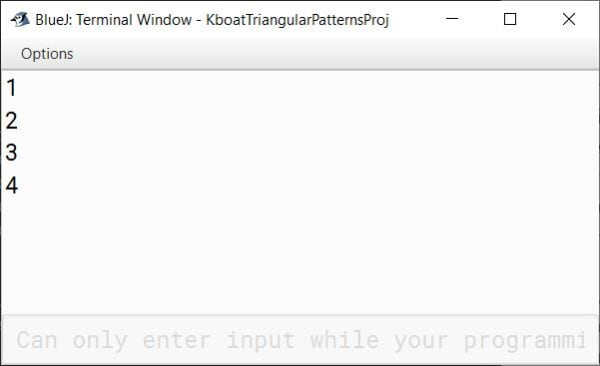See the value of i is 1, 2, 3, 4 which is same as the number of times our inner loop should execute per iteration of outer loop. So, I can write the inner loop and complete the program as shown below. (Output is provided below the program.)

```public class KboatPattern1
{
public static void main(String args[]) {
for (int i = 1; i <= 4; i++) {
for (int j = 1; j <= i; j++) {
System.out.print("* ");
}
System.out.println();
}
}
}```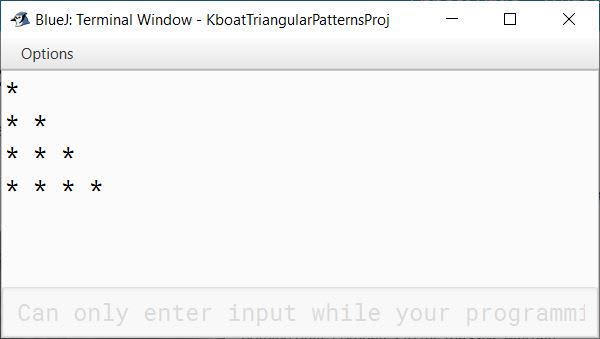Don’t worry if you are still a bit unsure about how this program is printing this pattern. The below dry run table will help you to understand the line-by-line execution of the program. (Underlined star in the output column denotes the star printed in that step.)

ijOutputRemarks
11*1st Iteration of outer for loop begins. i and j are initialized to 1.
21*
*
2nd Iteration of outer for loop begins. j starts from 1 again.
2*
* *
2nd Iteration of inner for loop prints second star of second row.
31*
* *
*
3rd Iteration of outer for loop begins. j starts from 1 again. Inner for loop prints first star of third row.
2*
* *
* *
2nd Iteration of inner for loop prints second star of third row.
3*
* *
* * *
3rd Iteration of inner for loop prints third star of third row.
41*
* *
* * *
*
4th Iteration of outer for loop begins. j starts from 1 again. Inner for loop prints first star of fourth row.
2*
* *
* * *
* *
2nd Iteration of inner for loop prints second star of fourth row.
3*
* *
* * *
* * *
3rd Iteration of inner for loop prints third star of fourth row.
4*
* *
* * *
* * * *
Final Output

## Pattern 2

Next pattern that we will solve is this:

* * * *
* * *
* *
*

We have just inverted the previous pattern. Let’s quickly analyse this pattern. It has 4 rows like before but this time the number of stars in each row starts from 4 and decreases with each row so that the last row has just one star. This tell us that the outer loop should still run for 4 iterations, but it should go in the reverse direction. So, in the outer loop i will start from 4, it should be greater than equal to 1 and as we are going from higher value to lower value it should decrease so i--`for (int i = 4; i >= 1; i--)`

The inner loop will be like before — `for (int j = 1; j <= i; j++)` . Inside inner loop we will print the star and then outside it we will write `System.out.println` to output the newline character. Below is the complete Java program along with its output in BlueJ.

```public class KboatPattern1
{
public static void main(String args[]) {
for (int i = 4; i >= 1; i--) {
for (int j = 1; j <= i; j++) {
System.out.print("* ");
}
System.out.println();
}
}
}```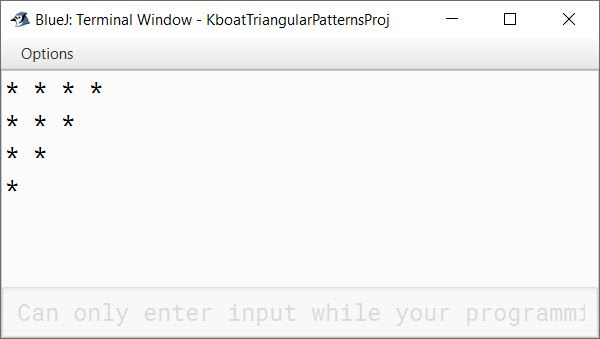Below table explains the dry run of the program outlining all the iterations of inner and outer loops:

ijOutputRemarks
41*1st Iteration of outer for loop begins. i starts from 4 and j starts from 1.
2* *2nd Iteration of inner for loop prints second star of first row.
3* * *3rd Iteration of inner for loop prints third star of first row.
4* * * *4th Iteration of inner for loop prints fourth star of first row.
31* * * *
*
2nd Iteration of outer for loop begins. i becomes 3, j starts from 1 again. Inner for loop prints first star of second row.
2* * * *
* *
2nd Iteration of inner for loop prints second star of second row.
3* * * *
* * *
3rd Iteration of inner for loop prints third star of second row.
21* * * *
* * *
*
3rd Iteration of outer for loop begins. i becomes 2, j starts from 1 again.
2* * * *
* * *
* *
2nd Iteration of inner for loop prints second star of third row.
11* * * *
* * *
* *
*
4th and final Iteration of outer for loop begins. Inner loop is executed only once and we get the final output.

## Pattern 3

Let’s solve this pattern now:

1
22
333
4444
55555

It has 5 rows, the number in each row are the row numbers and they are increasing from 1 to 5. So, the outer for loop will run from 1 to 5 like this — `for (int i = 1; i <= 5; i++)`

The inner loop will run from 1 to `i`. As the numbers in each row are the row numbers, we will print i inside the inner for loop. After the inner for loop, don’t forget to print a newline so that each row starts on a new line. Below is the complete Java program for this pattern along with its output in BlueJ:

```public class KboatPattern2
{
public static void main(String args[]) {
for (int i = 1; i <= 5; i++) {
for (int j = 1; j <= i; j++) {
System.out.print(i);
}
System.out.println(); // Prints newline so that each row starts on a new line
}
}
}```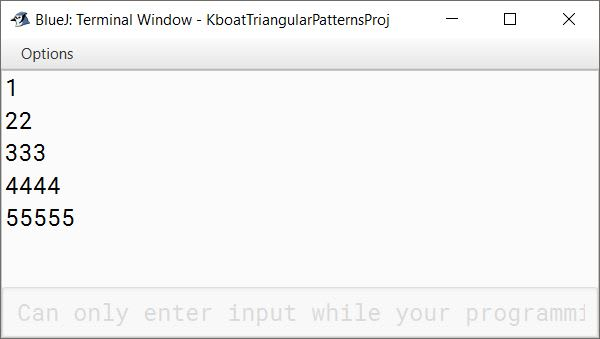Below table explains the dry run of the program:

ijOutputRemarks
1111st Iteration of outer for loop begins. i and j are initialized to 1.
211
2
2nd Iteration of outer for loop begins. j starts from 1 again.
21
22
2nd Iteration of inner for loop prints second 2 of second row.
311
22
3
3rd Iteration of outer for loop begins. j starts from 1 again. Inner for loop prints first 3 of third row.
21
22
33
2nd Iteration of inner for loop prints second 3 of third row.
31
22
333
3rd Iteration of inner for loop prints third 3 of third row.
411
22
333
4
4th Iteration of outer for loop begins. j starts from 1 again. Inner for loop prints first 4 of fourth row.
21
22
333
44
2nd Iteration of inner for loop prints second 4 of fourth row.
31
22
333
444
3rd Iteration of inner for loop prints third 4 of fourth row.
41
22
333
4444
4th Iteration of inner for loop prints fourth 4 of fourth row.
511
22
333
4444
5
5th Iteration of outer for loop begins. j starts from 1 again. Inner for loop prints first 5 of fifth row.
21
22
333
4444
55
2nd Iteration of inner for loop prints second 5 of fifth row.
31
22
333
4444
555
3rd Iteration of inner for loop prints third 5 of fifth row.
41
22
333
4444
5555
4th Iteration of inner for loop prints fourth 5 of fifth row.
51
22
333
4444
55555
5th Iteration of inner for loop prints fifth 5 of fifth row. After this, both the loops finish executing and we get our final output.

## Pattern 4

Next pattern is this:

1
12
123
1234
12345

The number of rows and number of terms in each row of this pattern are same as the previous pattern. The only difference is in what is getting printed in each row.

The code of this pattern will be similar to the previous pattern. The outer and inner for loops for this pattern will be the same as for the previous pattern. The only things that will change from the previous pattern is what we should print inside the inner for loop to get this pattern.

The first term of each row is 1, last term is the row number and terms inside the row are incrementing by 1. This sounds same as how j is incrementing. It starts at 1, goes till the row number and increments by 1 in each iteration. So, if we print j in the inner loop then we should get this pattern. Below is the complete Java program for this pattern along with its output in BlueJ:

```public class KboatPattern2
{
public static void main(String args[]) {
for (int i = 1; i <= 5; i++) {
for (int j = 1; j <= i; j++) {
System.out.print(j);
}
System.out.println(); // Prints newline so that each row starts on a new line
}
}
}```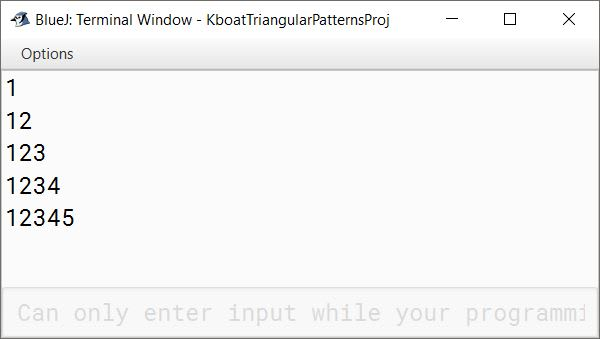Below table explains the dry run of the program:

ijOutputRemarks
1111st Iteration of outer for loop begins. i and j are initialized to 1.
211
1
2nd Iteration of outer for loop begins. j starts from 1 again.
21
12
2nd Iteration of inner for loop prints 2 of second row.
311
12
1
3rd Iteration of outer for loop begins. j starts from 1 again. Inner for loop prints 1 of third row.
21
12
12
2nd Iteration of inner for loop prints 2 of third row.
31
12
123
3rd Iteration of inner for loop prints 3 of third row.
411
12
123
1
4th Iteration of outer for loop begins. j starts from 1 again. Inner for loop prints 1 of fourth row.
21
12
123
12
2nd Iteration of inner for loop prints 2 of fourth row.
31
12
123
123
3rd Iteration of inner for loop prints 3 of fourth row.
41
12
123
1234
4th Iteration of inner for loop prints 4 of fourth row.
511
12
123
1234
1
5th Iteration of outer for loop begins. j starts from 1 again. Inner for loop prints 1 of fifth row.
21
12
123
1234
12
2nd Iteration of inner for loop prints 2 of fifth row.
31
12
123
1234
123
3rd Iteration of inner for loop prints 3 of fifth row.
41
12
123
1234
1234
4th Iteration of inner for loop prints 4 of fifth row.
51
12
123
1234
12345
5th Iteration of inner for loop prints 5 of fifth row. After this, both the loops finish executing and we get our final output.

## Pattern 5

Next pattern is this:

12345
2345
345
45
5

This is an inverted triangle, meaning number of terms in each row are decreasing as we come down. The first term of each row is the row number and last term is 5. If you see the first term of each row, it is increasing from 1 to 5. So, I will write outer loop like this — `for (int i = 1; i <= 5; i++)`

After this, in inner loop, I will start `j` from `i` as first term of each row is the row number. Condition will be `j <= 5` as last term is each row is 5 and then `j++`. Inside the inner loop, I will print `j`. And as always, add `System.out.println` after the inner loop. Below is the complete Java program for this pattern along with its output in BlueJ:

```public class KboatPattern3
{
public static void main(String args[]) {
for (int i = 1; i <= 5; i++) {
for (int j = i; j <= 5; j++) {
System.out.print(j);
}
System.out.println(); // Prints newline so that each row starts on a new line
}
}
}```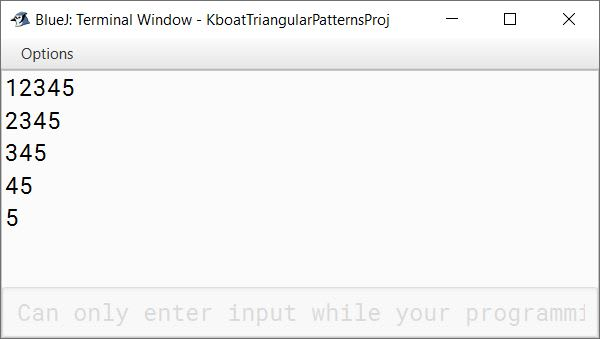The below table will help you to understand the line-by-line execution of the program. (Underlined number in the output column denotes the number printed in that step.)

ijOutputRemarks
1111st Iteration of outer for loop begins. i and j are initialized to 1.
2122nd Iteration of inner for loop prints 2 of the first row.
31233rd Iteration of inner for loop prints 3 of the first row.
412344th Iteration of inner for loop prints 4 of the first row.
5123455th Iteration of inner for loop prints 5 of the first row.
2212345
2
2nd Iteration of outer for loop begins. i becomes 2. j also becomes 2 as j is initialized to i.
312345
23
2nd Iteration of inner for loop prints 3 of the second row.
412345
234
3rd Iteration of inner for loop prints 4 of the second row.
512345
2345
4th Iteration of inner for loop prints 5 of the second row.
3312345
2345
3
3rd Iteration of outer for loop begins. i becomes 3. j also becomes 3 as j is initialized to i.
412345
2345
34
2nd Iteration of inner for loop prints 4 of the third row.
512345
2345
345
3rd Iteration of inner for loop prints 5 of the third row.
4412345
2345
345
4
4th Iteration of outer for loop begins. i becomes 4. j also becomes 4 as j is initialized to i.
512345
2345
345
45
2nd Iteration of inner for loop prints 5 of the fourth row.
5512345
2345
345
45
5
5th Iteration of outer for loop begins. i and j become 5. Inner for loop executes just once. After that we get our final pattern as output.

## Pattern 6

The next pattern that we will code is this:

97531
9753
975
97
9

This pattern is an inverted triangle with 5 rows. The first term of each row is 9 and last term of each row starts at 1 and goes till 9 increasing by 2 with each row. If I run my outer loop as shown below (i will start at 1, condition will be i less than equal to 9 and `i` will increment by 2 with each iteration) then `i` will give me the last term of each row.

```public class KboatPattern4
{
public static void main(String args[]) {
for (int i = 1; i <= 9; i += 2) {
}
}
}```

Coming to inner loop, all rows are starting with 9 so I will initialize j to 9. We already saw that i is giving the last term of each row so the condition of inner for loop will be `j >= i`. As the loop is going from higher value to lower value, so we need to decrement `j`. By how much should we decrement `j`? The difference between successive numbers in each row is 2 so we will decrement `j` by 2. Inside the inner loop I will print `j`. Lastly, I will add a `System.out.println` after the inner loop. The complete Java program for this pattern along with its output in BlueJ is given below:

```public class KboatPattern4
{
public static void main(String args[]) {
for (int i = 1; i <= 9; i += 2) {
for (int j = 9; j >= i; j -= 2) {
System.out.print(j);
}
System.out.println();
}
}
}```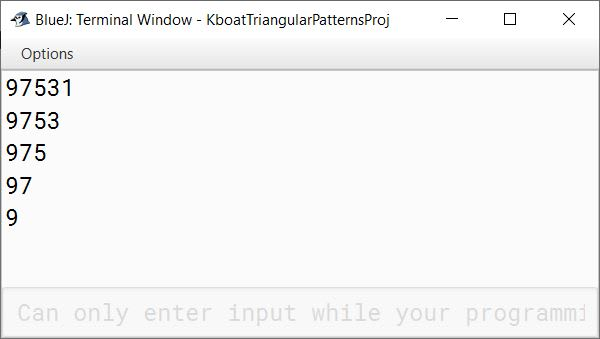Below table explains the dry run of the program:

ijOutputRemarks
1991st Iteration of outer for loop begins. i is initialized to 1 and j is initialized to 9. 1st Iteration of inner for loop prints the first 9.
7972nd Iteration of inner for loop prints 7 of the first row.
59753rd Iteration of inner for loop prints 5 of the first row.
397534th Iteration of inner for loop prints 3 of the first row.
1975315th Iteration of inner for loop prints 1 of the first row.
3997531
9
2nd Iteration of outer for loop begins. i becomes 3 and j starts from 9 again. 1st Iteration of inner for loop prints the 9 of the second row.
797531
97
2nd Iteration of inner for loop prints 7 of the second row.
597531
975
3rd Iteration of inner for loop prints 5 of the second row.
397531
9753
4th Iteration of inner for loop prints 3 of the second row.
5997531
9753
9
3rd Iteration of outer for loop begins. i becomes 5 and j starts from 9 again. 1st Iteration of inner for loop prints the 9 of the third row.
797531
9753
97
2nd Iteration of inner for loop prints 7 of the third row.
597531
9753
975
3rd Iteration of inner for loop prints 5 of the third row.
7997531
9753
975
9
4th Iteration of outer for loop begins. i becomes 7 and j starts from 9 again. 1st Iteration of inner for loop prints the 9 of the fourth row.
797531
9753
975
97
2nd Iteration of inner for loop prints 7 of the fourth row.
9997531
9753
975
97
9
5th Iteration of outer for loop begins and i becomes 9. j starts from 9 again. 1st Iteration of inner for loop prints the 9 of the fifth row. With this our pattern is complete and both loops stop executing.